# 《Lua游戏AI开发指南》一2.10　智能体的属性

+关注继续查看

## 2.10.1　朝向

1．前向轴

local forwardVector = agent:GetForward();
Agent.SetForward(agent, forwardVector);


2．左向轴

local leftVector = agent:GetLeft();


3．向上的轴

local upVector = agent:GetUp();


### 2.10.2　定位

local positionVector = agent:GetPosition();
agent:SetPosition(positionVector);


### 2.10.3　大小

1．高度

local height = agent:GetHeight();
agent:SetHeight(height);


2．半径

local radius = agent:GetRadius();


### 2.10.4　物理

1．质量

local mass = agent:GetMass();
agent:SetMass(mass);


2．最大作用力

local maxForce = agent:GetMaxForce();
agent:SetMaxForce(maxForce);


3．最大速率

local maxSpeed = agent:GetMaxSpeed();
agent:SetMaxSpeed(maxSpeed);


4．速率

local speed = agent:GetSpeed();
agent:SetSpeed(speed);


5．速度

local velocityVector = agent:GetVelocity();
agent:SetVelocity(velocityVector);


### 2.10.5　知识

1．目标

local targetVector = agent:GetTarget();
agent:SetTarget(targetVector);


2．目标半径

local targetRadius = agent:GetTargetRadius();


3．路径

local pathTable = agent:GetPath();
local hasPath = agent:HasPath();

local path = {
Vector.new(0, 0, 0),
Vector.new(10, 0, 10),
Vector.new(0, 0, 10) };

agent:SetPath(path, cylic);


### 2.10.6　智能体的移动

1．质量

2．速率

3．速度

4．加速度

5．力

Newtons = kilograms * meters / second 2


### 2.10.7　智能体转向力

1．探索

local seekForce = agent:ForceToPosition(destination);


2．对智能体应用转向力

AgentUtilities.lua:

function AgentUtilities_ApplyPhysicsSteeringForce (
agent, steeringForce, deltaTimeInSeconds)

-- Ignore very weak steering forces.
if (Vector.LengthSquared(steeringForce) < 0.1) then
return;
end

--Agents with 0 mass are immovable.
if (agent:GetMass() <= 0) then
return;
end

-- Zero out any steering in the y direction
steeringForce.y = 0;

-- Maximize the steering force, essentially forces the agent
-- to max acceleration.
steeringForce =
Vector.Normalize(steeringForce) * agent:GetMaxForce();

--Apply force to the physics representation.
agent:ApplyForce(steeringForce);

-- Newtons(kg*m/s^2) divided by mass(kg) results in
-- acceleration(m/s^2).

local acceleration = steeringForce / agent:GetMass();

-- Velocity is measured in meters per second(m/s).
local currentVelocity = agent:GetVelocity();

-- Acceleration(m/s^2) multiplied by seconds results in
-- velocity(m/s).
local newVelocity =
currentVelocity + (acceleration * deltaTimeInSeconds);

-- Zero out any pitch changes to keep the Agent upright.
-- NOTE: This implies that agents can immediately turn in any
-- direction.
newVelocity.y = 0;

-- Point the agent in the direction of movement.
agent:SetForward(newVelocity);
end


3．限制智能体的水平速率

AgentUtilities.lua:

function AgentUtilities_ClampHorizontalSpeed(agent)
local velocity = agent:GetVelocity();
-- Store downward velocity to apply after clamping.
local downwardVelocity = velocity.y;

-- Ignore downward velocity since Agents never apply downward
-- velocity themselves.
velocity.y = 0;

local maxSpeed = agent:GetMaxSpeed();
local squaredSpeed = maxSpeed * maxSpeed;

-- Using squared values avoids the cost of using the square
-- root when calculating the magnitude of the velocity vector.
if (Vector.LengthSquared(velocity) > squaredSpeed) then
local newVelocity =
Vector.Normalize(velocity) * maxSpeed;

-- Reapply the original downward velocity after clamping.
newVelocity.y = downwardVelocity;

agent:SetVelocity(newVelocity);
end
end


4．创建一个探索智能体

Agent.lua:

require "AgentUtilities";

function Agent_Initialize(agent)

...

-- Assign a default target and acceptable target radius.
agent:SetTarget(Vector.new(50, 0, 0));
end

function Agent_Update(agent, deltaTimeInMillis)
local destination = agent:GetTarget();
local deltaTimeInSeconds = deltaTimeInMillis / 1000;
local seekForce = agent:ForceToPosition(destination);
local position = agent:GetPosition();

-- Apply seeking force.
AgentUtilities_ApplyForce(
agent, seekForce, deltaTimeInSeconds);
AgentUtilities_ClampHorizontalSpeed(agent);

-- Calculate the position where the Agent touches the ground.
agent:GetPosition() -
Vector.new(0, agent:GetHeight()/2, 0);

-- If the agent is within the target radius pick a new
-- random position to move to.

-- New target is within the 100 meter squared movement
-- space.
local target = agent:GetTarget();
target.x = math.random(-50, 50);
target.z = math.random(-50, 50);

agent:SetTarget(target);
end

-- Draw debug information for target and target radius.
Core.DrawCircle(
Core.DrawLine(position, destination, Vector.new(0, 1, 0));

-- Debug outline representing the space the Agent can move
-- within.
Core.DrawSquare(Vector.new(), 100, Vector.new(1, 0, 0));
end


src/my_sandbox/script/Agent.lua to
src/my_sandbox/ script/SeekingAgent.lua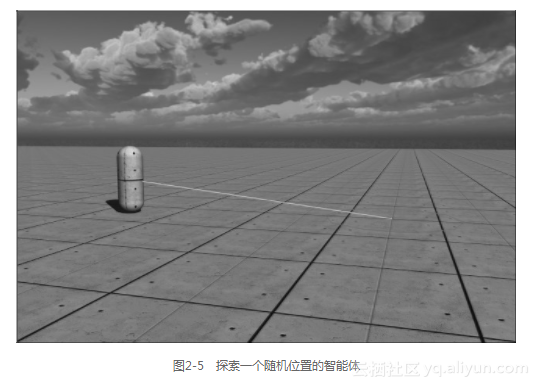5．追逐

src/my_sandbox/script/PursuingAgent.lua


PursuingAgent.lua:

require "AgentUtilities";

local enemy;

function Agent_Cleanup(agent)
end

function Agent_HandleEvent(agent, event)
end

function Agent_Initialize(agent)
AgentUtilities_CreateAgentRepresentation(

-- Assign an acceptable target radius.
-- Randomly assign a position to the agent.
agent:SetPosition(Vector.new(
math.random(-50, 50),
0,
math.random(-50, 50)));

local agents = Sandbox.GetAgents(agent:GetSandbox());

-- Find the first valid agent and assign the agent as an
-- enemy.
for index = 1, #agents do
if (agents[index] ~= agent) then
enemy = agents[index];
agent:SetTarget(enemy:GetPosition());
break;
end
end

-- Make the pursuing Agent slightly slower than the enemy.
agent:SetMaxSpeed (enemy:GetMaxSpeed() * 0.8);
end

function Agent_Update(agent, deltaTimeInMillis)
-- Calculate the future position of the enemy agent.
agent:SetTarget (enemy:PredictFuturePosition(1));

local destination = agent:GetTarget();
local deltaTimeInSeconds = deltaTimeInMillis / 1000;
local seekForce = agent:ForceToPosition(destination);
local position = agent:GetPosition();

-- Apply seeking force to the predicted position.
AgentUtilities_ApplyForce(
agent, seekForce, deltaTimeInSeconds);
AgentUtilities_ClampHorizontalSpeed(agent);

-- Draw debug information for target and target radius.
Core.DrawCircle(
Core.DrawLine(position, destination, Vector.new(0, 1, 0));
end


Sandbox.lua:

function Sandbox_Initialize(sandbox)

...

Sandbox.CreateAgent(sandbox, "SeekingAgent.lua");
Sandbox.CreateAgent(sandbox, "PursuingAgent.lua");
end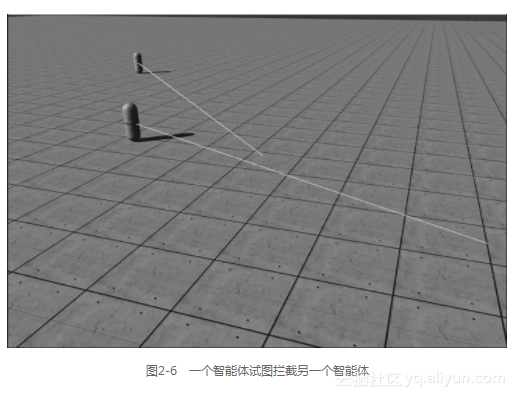6．逃跑

local forceToFlee = agent:ForceToFleePosition(position);


7．躲避

local forceToEvade = agent:ForceToFleePosition(
enemy:PredictFuturePosition(timeInSeconds));


8．漫游

local forceToWander = agent:ForceToWander(deltaTimeInMillis);


9．目标速率

local forceToSpeed = agent:ForceToTargetSpeed(targetSpeed);


10．路径追踪

11．创建一个路径追踪智能体

src/my_sandbox/script/PathingAgent.lua


PathingAgent.lua:

require "AgentUtilities";
require "DebugUtilities";

function Agent_Initialize(agent)

AgentUtilities_CreateAgentRepresentation(

-- Randomly assign a position to the agent.
agent:SetPosition(Vector.new(
math.random(-50, 50),
0,
math.random(-50, 50)));
end

function Agent_Update(agent, deltaTimeInMillis)
local deltaTimeInSeconds = deltaTimeInMillis / 1000;

-- Force to continue moving along the path, can cause the
-- agent to veer away from the path.
local followForce = agent:ForceToFollowPath(1.25);

-- Force to move to the closest point on the path.
local stayForce = agent:ForceToStayOnPath(1);

-- Slight deviation force to alleviate bumping other pathing
-- agents.
local wanderForce = agent:ForceToWander(deltaTimeInMillis);

-- Sum steering forces using scalars.
local totalForces =
Vector.Normalize(followForce) +
Vector.Normalize(stayForce) * 0.25 +
Vector.Normalize(wanderForce) * 0.25;

local targetSpeed = 3;

-- Accelerate pathing agents to a minimum speed.
if (agent:GetSpeed() < targetSpeed) then
local speedForce = agent:ForceToTargetSpeed(targetSpeed);
totalForces = totalForces + Vector.Normalize(speedForce);
end

-- Apply the summation of all forces.
AgentUtilities_ApplyPhysicsSteeringForce(
agent, totalForces, deltaTimeInSeconds);
AgentUtilities_ClampHorizontalSpeed(agent);

-- Draw the agent's path as a looping path.
DebugUtilities_DrawPath (agent:GetPath(), true);
end


Agent_Update函数添加了一些新的代码，展示了如何把两个转向力加到一起。ForceToFollowPath和ForeceToStayOnPath都以一个与StayOnPath力相关的较小的权重加到一起。寻路智能体中还添加了一个ForceToTargetSpeed函数以确保它的速度不会小于一个最小速度。

Sandbox.lua:

-- Default path to assign to path following agents.
local path = {
Vector.new(0, 0, 0),
Vector.new(30, 0, 0),
Vector.new(30, 0, 50),
Vector.new(-30, 0, 0),
Vector.new(-30, 0, 20)};

function Sandbox_Initialize(sandbox)

...

for i=1, 20 do
local agent = Sandbox.CreateAgent(
sandbox, "PathingAgent.lua");

-- Assign the same path to every agent.
agent:SetPath(path, true);

-- Randomly vary speeds to allow agents to pass one
-- another.
local randomSpeed = math.random(
agent:GetMaxSpeed() * 0.85,
agent:GetMaxSpeed() * 1.15);

agent:SetMaxSpeed(randomSpeed);
end
end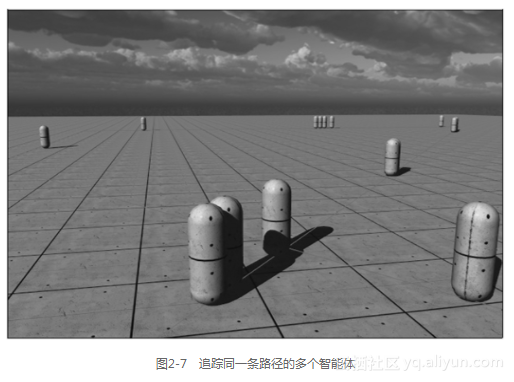### 2.10.8　规避

1．避免碰撞

local avoidAgentForce =
agent:ForceToAvoidAgents(minTimeToCollision);


2．规避障碍物

local avoidObjectForce =
agent:ForceToAvoidObjects(minTimeToCollision);


### 2.10.9　规避障碍物和其他智能体

SeekingAgent.lua

function Agent_Update(agent, deltaTimeInMillis)
local destination = agent:GetTarget();
local deltaTimeInSeconds = deltaTimeInMillis / 1000;
local avoidAgentForce = agent:ForceToAvoidAgents(1.5);
local avoidObjectForce = agent:ForceToAvoidObjects (1.5);
local seekForce = agent:ForceToPosition(destination);
local position = agent:GetPosition();
local avoidanceMultiplier = 3;

-- Sum all forces and apply higher priority to avoidance
-- forces.
local steeringForces =
seekForce +
avoidAgentForce * avoidanceMultiplier +
avoidObjectForce * avoidanceMultiplier;

AgentUtilities_ApplyForce(
agent, steeringForces, deltaTimeInSeconds);

...

end

Sandbox.lua:

require "DebugUtilities";

function Sandbox_Update(sandbox, deltaTimeInMillis)

-- Grab all Sandbox objects, not including agents.
local objects = Sandbox.GetObjects(sandbox);

-- Draw debug bounding sphere representations for objects with
-- mass.
DebugUtilities_DrawDynamicBoundingSpheres(objects);
end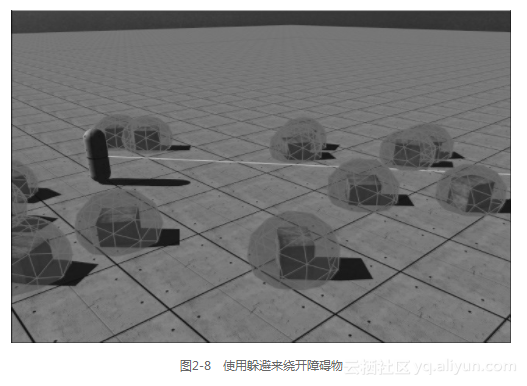### 2.10.10　群组移动

1．对齐

local forceToAlign =
agent:ForceToSeparate(maxDistance, maxAngle, agents);


2．聚拢

local forceToCombine =
agent:ForceToCombine(maxDistance, maxAngle, agents);


3．分离

local forceToSeparate =

   agent:forceToSeparate(minDistance, maxAngle, agents);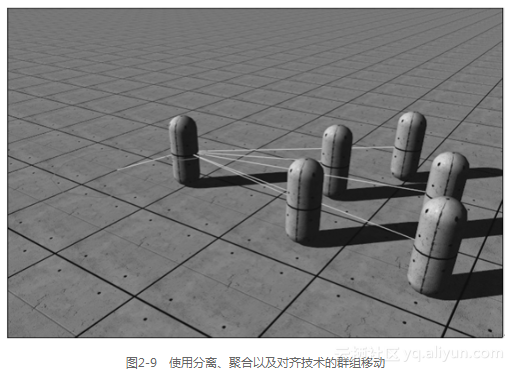### 2.10.11　创建一群追随者

src/my_sandbox/script/FollowerAgent.lua

FollowerAgent.lua:

require "AgentUtilities";

function Agent_Initialize(agent)
AgentUtilities_CreateAgentRepresentation(

-- Randomly assign a position to the agent.
agent:SetPosition(Vector.new(
math.random(-50, 50), 0, math.random(-50, 50)));

-- Assign the first valid agent as the leader to follow.
local agents = Sandbox.GetAgents(agent:GetSandbox());
for index = 1, #agents do
if (agents[index] ~= agent) then
break;
end
end
end

function Agent_Update(agent, deltaTimeInMillis)
local deltaTimeInSeconds = deltaTimeInMillis / 1000;
local sandboxAgents = Sandbox.GetAgents(agent:GetSandbox());

-- Calculate a combining force so long as the leader stays
-- within a 100 meter range from the agent, and has less than
-- 180 degree difference in forward direction.
local forceToCombine =
Agent.ForceToCombine(agent, 100, 180, { leader } );

-- Force to stay away from other agents that are closer than
-- 2 meters and have a maximum forward degree difference of
-- less than 180 degrees.
local forceToSeparate =
Agent.ForceToSeparate(agent, 2, 180, sandboxAgents );

-- Force to stay away from getting too close to the leader if
-- within 5 meters of the leader and having a maximum forward
-- degree difference of less than 45 degrees.
local forceToAlign =
Agent.ForceToSeparate(agent, 5, 45, { leader } );

-- Summation of all separation and cohesion forces.
local totalForces =
forceToCombine + forceToSeparate * 1.15 + forceToAlign;

-- Apply all steering forces.
AgentUtilities_ApplyPhysicsSteeringForce(
agent, totalForces, deltaTimeInSeconds);
AgentUtilities_ClampHorizontalSpeed(agent);

local position = agent:GetPosition();

-- Draw debug information for target and target radius.
Core.DrawCircle(
position, 1, Vector.new(1, 1, 0));
Core.DrawCircle(
Core.DrawLine(position, destination, Vector.new(0, 1, 0));
end


Sandbox.lua:

function Sandbox_Initialize(sandbox)

...

-- Create a pursuing agent to follow the seeking agent.
Sandbox.CreateAgent(sandbox, "PursuingAgent.lua");

-- Create a group of followers that follow the seeking agent.
for i=1, 5 do
Sandbox.CreateAgent(sandbox, "FollowerAgent.lua");
end

...

end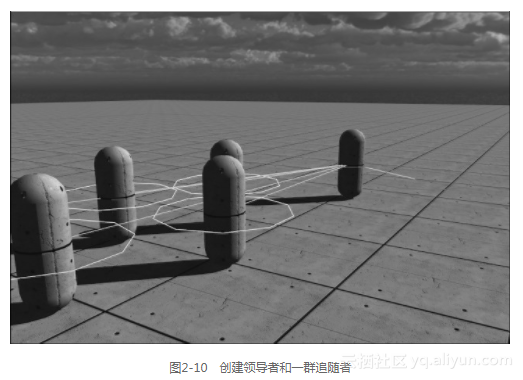### 2.10.12　转向力合计

1．加权合计

2．基于优先级的力|
7天前
|

Unity——游戏AI实例
Unity——游戏AI实例
16 0
|
7天前
|

15 0
|
3月前
|

81 0
|
3月前
|

【Python】利用tkinter开发AI对战井字棋游戏
【Python】利用tkinter开发AI对战井字棋游戏
85 0
|
4月前
|

|
5月前
|

ChatGPT唤醒AI游戏？
ChatGPT唤醒AI游戏？
100 0
|
6月前
|

Level Up！真格 AI 游戏黑客松等你来战
Level Up！真格 AI 游戏黑客松等你来战
276 0
|
6月前
|

57 0
|
6月前
|

258 0
|
6月前
|

61 0